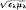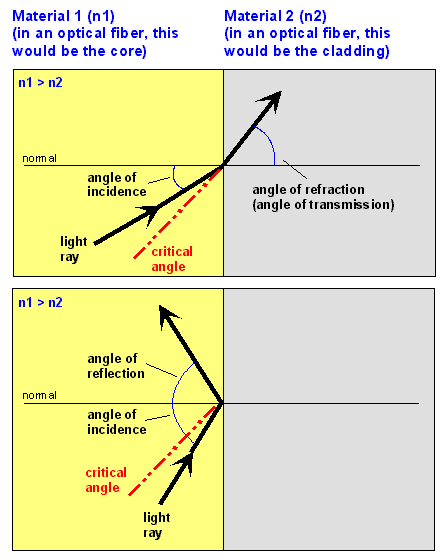refractive index

Also found in: Dictionary, Thesaurus, Medical, Legal, Financial, Acronyms, Wikipedia.
Related to refractive index: Total internal reflection, refractometer

refractive index

(ri-frak -tiv) (index of refraction) Symbol: n . The ratio of the speed of light, c , in a vacuum to the speed of light in a given medium. This is the absolute refractive index; it is always greater than one. The relative refractive index is the ratio of the speeds of light in two given media. The refractive index of a medium depends on the wavelength of the refracted wave: with light waves, n increases as the wavelength decreases, i.e. blue light is refracted (bent) more than red light. See also refraction.
Collins Dictionary of Astronomy © Market House Books Ltd, 2006
The following article is from The Great Soviet Encyclopedia (1979). It might be outdated or ideologically biased.

Refractive Index

The relative refractive index of two media is the dimensionless ratio n21 of the velocity of the propagation of light (less frequently, of radio waves) in the first medium (v1) to that in the second medium (v2); thus, n21 = v1/v2. At the same time, the relative refractive index is the ratio of the sines of the angle of incidence a and the angle of refraction β at the interface between the two media: n21 = sin α/sin β. If the first medium is a vacuum (in which the speed of light is c ≃ 3 × 1010 cm/sec), the refractive index of the medium relative to the vacuum is called the absolute refractive index: n = c/v. The relative refractive index is the ratio between the absolute refractive indexes of the two media: n21 = n2/n1.

The refractive index depends on the wavelength λ (frequency v) of the radiation (seeDISPERSION OF LIGHT). The absolute refractive index is related to the dielectric constant ∊ and magnetic permeability μ of the medium by the equation =(∊ and μ are also functions of λ). The absolute refractive index of a medium is determined by the polarizability of its component particles, as well as by the structure of the medium and its state of aggregation (seeCLAUSIUS-MOSSOTTI EQUATION, LORENTZ-LORENZ FORMULA, AND ). In the case of mediums characterized by optical anisotropy, whether natural or induced, the refractive index depends on the radiation’s direction of propagation and its polarization. Many crystals are typical anisotropic media (seeCRYSTAL OPTICS). Media that absorb radiation are described by a complex refractive index ñ = n(1 + ik), where the term containing only n usually corresponds to the transmission of light without scattering and κ = /4π describes absorption (k is the absorption coefficient of the medium). (See.)

In the range of visible light, the refractive index varies from 1.3 to 4.0 for weakly absorbing transparent solids. For liquids it ranges from 1.2 to 1.9, and for gases under normal conditions from 1.000035 (He) to 1.000702 (Xe).

refractive index

[ri′frak·tiv ‚in‚deks]
(optics)
McGraw-Hill Dictionary of Scientific & Technical Terms, 6E, Copyright © 2003 by The McGraw-Hill Companies, Inc.

refractive index

A property of a material that changes the speed of light, computed as the ratio of the speed of light in a vacuum to the speed of light through the material. When light travels at an angle between two different materials, their refractive indices determine the angle of transmission (refraction) of the light beam. In general, the refractive index varies based on the frequency of the light as well, thus different colors of light travel at different speeds. High intensities also can change the refractive index.

The refractive index of a vacuum is 1.0, and air is a tiny fraction greater than 1.0. The higher the index, the slower the speed of light through the medium, because the speed through the material is the speed of light (c) over the refractive index (n), thus speed = c/n. Following are common refractive indices. See fiber optics glossary.

RefractiveMaterial          Index (n)

Vacuum            1.0

Air               1.0**

Water             1.33

Glass             1.45-1.48

Lithium niobate   2.25

Gallium arsenide  3.35

Silicon           3.5

Germanium         4.0

** = air is a tiny franction
greater than 1.0

Refractive IndicesWhen light travels at an angle between two materials, light bends according to their refractive indices. In order to reflect, light must be on the wider side of the critical angle.
Copyright © 1981-2019 by The Computer Language Company Inc. All Rights reserved. THIS DEFINITION IS FOR PERSONAL USE ONLY. All other reproduction is strictly prohibited without permission from the publisher.
References in periodicals archive ?
The sensing sensitivity is defined as the shift of resonance wavelength versus the change in surrounding refractive index. If the refractive index value is increased by higher concentration of NaCl solutions, the LMR peak shifts to higher wavelengths.
By considering the thickness of the structure as 24 mm, the maximum refractive index "[n.sub.0]" and minimum refractive index "n(x)" values are found to be 1.82 and 1.276, respectively.
where [n.sub.s] and [n.sub.l] refer to the refractive index of the solid and liquid, respectively; [k.sub.1] refers to the coefficient that is modified by the size, shape, and distribution of the solid particle; [k.sub.2] refers to the coefficient that is modified by the shape and volume fraction of the solid particle; d refers to the transmitted thickness or viewing depth; and [[lambda].sub.m] refers to the matching wavelength at which the solid and liquid refractive indexes are matched.
"High-sensitive double-hole defect refractive index sensor based on 2-D photonic crystal", IEEE Sensor Journal, 13.
To measure the refractive index of the prepared Ti[O.sub.2] NMO over the wafer, a spectroscopic ellipsometry (HORIBA JOBIN YVOIN) was employed.
The value of the coupling coefficient depends on the mechanical tension applied to the fiber and on the laser power, as both parameters affect the refractive index modulation in both core and cladding.
(11.) Liu, Y, Lii, C, Li, M, Zhang, L, Yang, B, "High Refractive Index Organic-Inorganic Hybrid Coatings with Ti[O.sub.2] Nanocrystals." Colloids Surf.
In sample A, 5mA cm2 was applied for 155 seconds to produce layers with low porosity corresponding to high refractive index (denoted by H).
The propagation can be calculated as if the medium was isotropic with tunable refractive index because all the power couples into the extraordinary ray for this configuration.
The refractive index of extraordinary light and ordinary light of LC 5CB at 25[degrees]C are around 1.77 and 1.58 in the frequency range of 0.2 to 1.0 THz, respectively [25, 26] where 0 is determined by:
For many years, the standard method for assessing wash bath concentration has been refractive index. Its primary instrument, the refractometer, is inexpensive and easy to use, and the process is fast.

Site: Follow: Share:
Open / Close